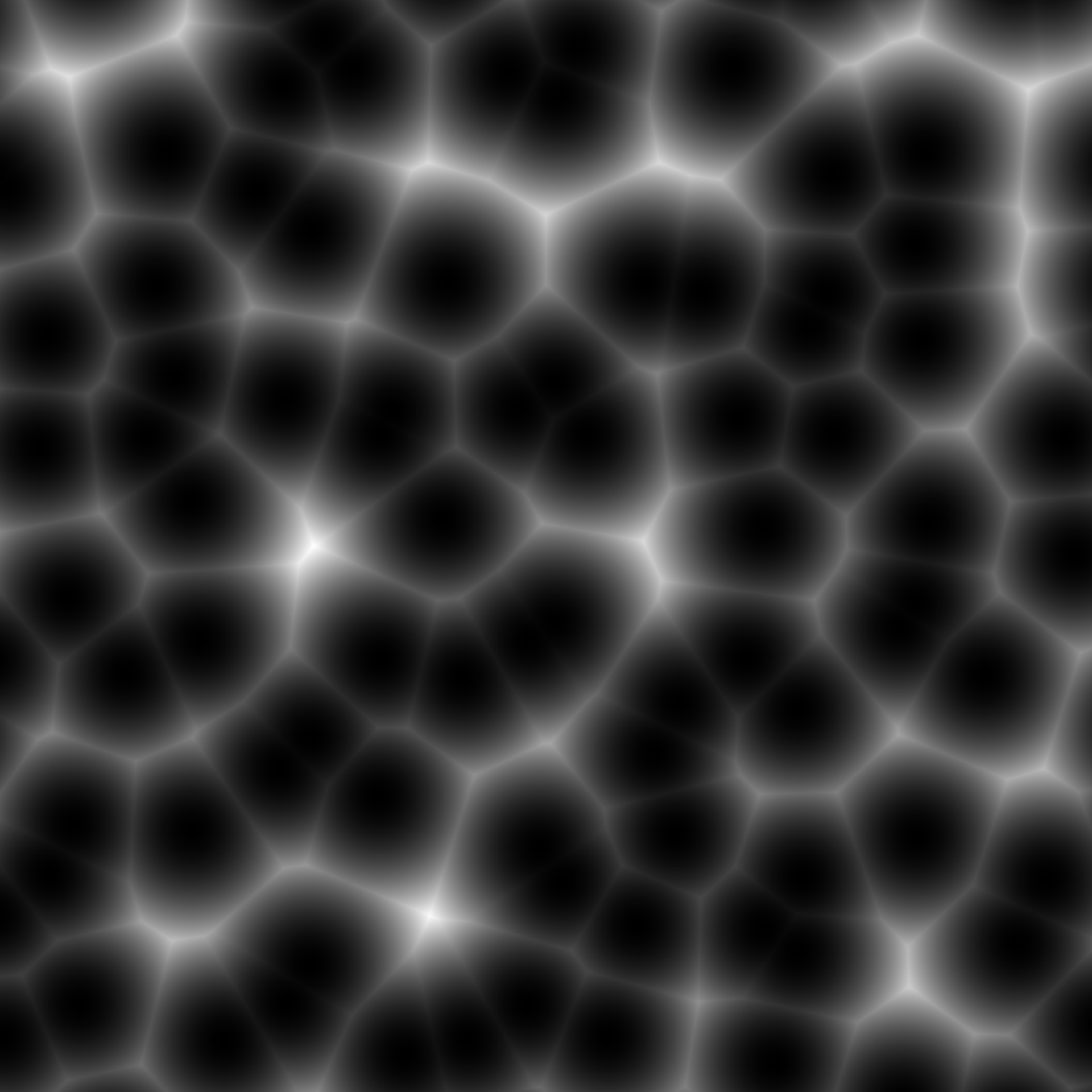Worley noise, sometimes called cell (or cellular) noise, is quite distinct due to it's kinship to voronoi tesselation. It is created by sampling random points in space and then for any point in space measure the distance to the closest point. The noise can be modified further by changing either the distance measure or by combining multiple distances. The noise algorithm was developed by Steven Worley in 1996 and has been used to simulated water and stone textures among other things.

noise_worley(
dim,
frequency = 0.01,
distance = "euclidean",
fractal = "none",
octaves = 3,
lacunarity = 2,
gain = 0.5,
value = "cell",
distance_ind = c(1, 2),
jitter = 0.45,
pertubation = "none",
pertubation_amplitude = 1
)

gen_worley(
x,
y = NULL,
z = NULL,
frequency = 1,
seed = NULL,
distance = "euclidean",
value = "cell",
distance_ind = c(1, 2),
jitter = 0.45,
...
)

## Arguments

dim

The dimensions (height, width, (and depth)) of the noise to be generated. The length determines the dimensionality of the noise.

frequency

Determines the granularity of the features in the noise.

distance

The distance measure to use, either 'euclidean' (default), 'manhattan', or 'natural' (a mix of the two)

fractal

The fractal type to use. Either 'none', 'fbm' (default), 'billow', or 'rigid-multi'. It is suggested that you experiment with the different types to get a feel for how they behaves.

octaves

The number of noise layers used to create the fractal noise. Ignored if fractal = 'none'. Defaults to 3.

lacunarity

The frequency multiplier between successive noise layers when building fractal noise. Ignored if fractal = 'none'. Defaults to 2.

gain

The relative strength between successive noise layers when building fractal noise. Ignored if fractal = 'none'. Defaults to 0.5.

value

The noise value to return. Either

• 'value' (default) A random value associated with the closest point

• 'distance' The distance to the closest point

• 'distance2' The distance to the nth closest point (n given by distance_ind)

• 'distance2add' Addition of the distance to the nth and mth closest point given in distance_ind

• 'distance2sub' Substraction of the distance to the nth and mth closest point given in distance_ind

• 'distance2mul' Multiplication of the distance to the nth and mth closest point given in distance_ind

• 'distance2div' Division of the distance to the nth and mth closest point given in distance_ind

distance_ind

Reference to the nth and mth closest points that should be used when calculating value.

jitter

The maximum distance a point can move from its start position during sampling of cell points.

pertubation

The pertubation to use. Either 'none' (default), 'normal', or 'fractal'. Defines the displacement (warping) of the noise, with 'normal' giving a smooth warping and 'fractal' giving a more eratic warping.

pertubation_amplitude

The maximal pertubation distance from the origin. Ignored if pertubation = 'none'. Defaults to 1.

x, y, z

Coordinates to get noise value from

seed

The seed to use for the noise. If NULL a random seed will be used

...

ignored

## Value

For noise_worley() a matrix if length(dim) == 2 or an array if length(dim) == 3. For gen_worley() a numeric vector matching the length of the input.

## Examples

# Basic use
noise <- noise_worley(c(100, 100))

plot(as.raster(normalise(noise)))# Using the generator and another value metric
grid <- long_grid(seq(1, 10, length.out = 1000), seq(1, 10, length.out = 1000))
grid$noise <- gen_worley(grid$x, grid\$y, value = 'distance')
plot(grid, noise)